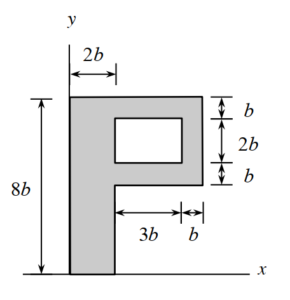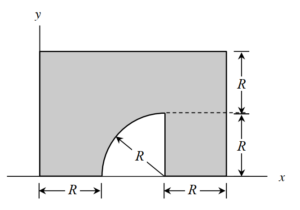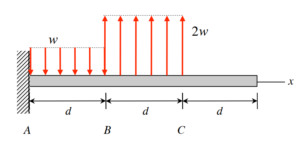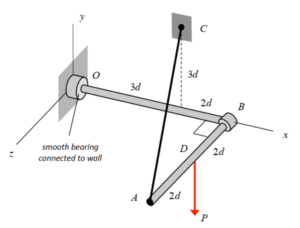# HOMEWORK 15.B - FA 23

 Problem statement Solution videoPlease post questions here on the homework, and take time to answer questions posted by others. You can learn both ways.

# HOMEWORK 15.A - FA 23

 Problem statement Solution videoPlease post questions here on the homework, and take time to answer questions posted by others. You can learn both ways.

# HOMEWORK 14.A - FA 23

 Problem statement Solution videoPlease post questions here on the homework, and take time to answer questions posted by others. You can learn both ways.

# HOMEWORK 14.B - FA 23

 Problem statement Solution videoPlease post questions here on the homework, and take time to answer questions posted by others. You can learn both ways.

# HOMEWORK 13.B - FA 23

 Problem statement Solution videoPlease post questions here on the homework, and take time to answer questions posted by others. You can learn both ways.

# HOMEWORK 13.A - FA 23

 Problem statement Solution videoPlease post questions here on the homework, and take time to answer questions posted by others. You can learn both ways.

# HOMEWORK 12.B - FA 23

 Problem statement Solution videoPlease post questions here on the homework, and take time to answer questions posted by others. You can learn both ways.

# HOMEWORK 12.A - FA 23

 Problem statement Solution videoPlease post questions here on the homework, and take time to answer questions posted by others. You can learn both ways.

# HOMEWORK 11.B - FA 23

 Problem statement Solution video